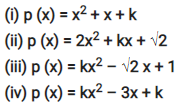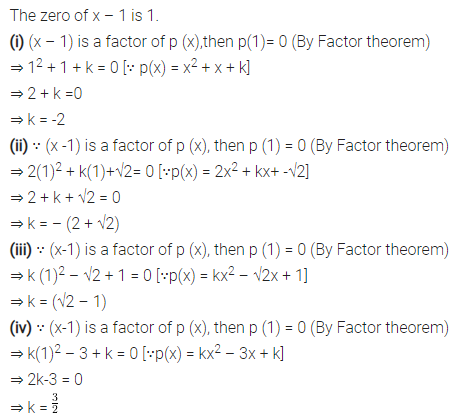# Find the value of k, if x – 1 is a factor of p (x)

Find the value of k, if x – 1 is a factor of p (x) in each of the following cases